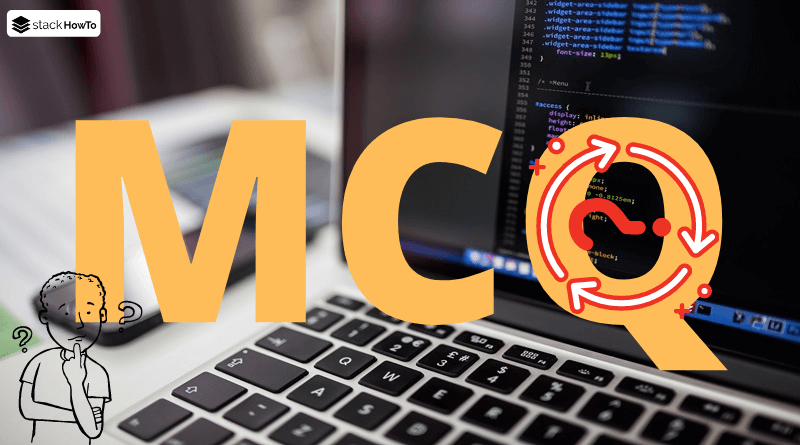MCQ

# JavaScript MCQs – Multiple Choice Questions and Answers – Part 3

Multiple choice questions and answers (MCQs) on JavaScript to prepare for exams, tests, and certifications. These questions are taken from a real written exam and some parts are taken from an interview. So you will find questions on basic techniques such as variables, functions, loops, objects, and more. This quiz will easily prepare anyone to pass their online test.

###### 1. How to declare an array of 10 elements in JavaScript?

A `arr = new Array(10)`

B `var arr`

C `var arr = array(10)`

D `var arr(10)`

A

###### 2. How to get a random number between 5 and 9 inclusive?

A `Math.floor((Math.random() * 5) + 4);`

B `Math.floor((Math.random() * 4) + 4);`

C `Math.floor((Math.random() * 4) + 5);`

D `Math.floor((Math.random() * 5) + 5);`

D

###### 3. To check if three variables are equal, we are going to use_____?

A `X = Y = Z`

B `(X == B) && (Y == Z)`

C `(X = B) && (Y = Z)`

D `(X == B) & (Y == Z)`

B

###### 4. How many parameters can be passed to a function?

A Any

B As much as you want

C One for each argument

D One argument

C
Example:

```function add(a, b) {
return a + b;
}

###### 5. Which of these parameters is not valid?

A text

B a variable

C an operator

D a number

C
We can’t pass an operator as a parameter to a function.

###### 6. How to detect the name of the client’s browser?

A navigator.appName

B browser.name

C client.navName

D window.appName

A
appName Returns the name of the browser. Example:

```if(navigator.appName == "Netscape")
{
}```

###### 7. If `str = "VWXYZ"`, what returns `str.charAt(3)`?

A X

B Y

C Z

D false

B
The method charAt() returns the character at the specified index in a string. The index of the first character is 0.

###### 8. The following statement `A ? B : C` is equivalent to ______?

A `if (A) {B; C}`

B `if (A != B) C`

C `if (A == B) C`

D `if (A) {B} else {C}`

D

A <

B >

C =

D !=

C

###### 10. Which event is specific to the keyboard?

A onkeypress

B onkeydown

C onclick

D onfocus

A
The event “onkeypress” occurs when the user presses a key (on the keyboard).MCQPractice competitive and technical Multiple Choice Questions and Answers (MCQs) with simple and logical explanations to prepare for tests and interviews.Read More